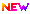High Impact Factor : 4.396| Submit Manuscript Online|

## Analysis of Plates for Building Construction with Different Shapes by Classical Plate Theory and NavierÃ¢â‚¬â„¢s Theory

### Author(s):

Rajesh Kumar Jha , NRI Institute of Research & Technology; Prof. Thakur Ramji Ram, NRI Institute of Research & Technology; Prof. Kamlesh Saha, NRI IRT, BHOPAL, M.P.

### Keywords:

Plate Theories, High Order Theory, Laminate, Composite, FGM, laminated composites, shear deformation plate theory, bending, vibration, buckling

### Abstract

The analysis of isotropic rectangular and square plates using Classical Plate Theory and NavierÃ¢â‚¬â„¢s Theory. In case of rectangular plates variation is done for length width ratio and thickness. And in case of square plate variation is done for thickness. The symmetric bending of Rectangular and Square plate is considered in the present study. Plates are analyzed for uniformly distributed load and concentrated point load in the present study. In this research work, the effect of varying thickness of the plate on its deflection and bending stress is studied. So, the key point of research work is thickness variation of plate. Once deflection is obtained by using Classical Plate Theory, bending moments and bending stresses are easily calculated by usual relations. This study discusses the elastic equilibrium problems of rectangular and square thin plates of varying thickness and simply supported on all four sides by linear and nonlinear theory. Using the Classical and NavierÃ¢â‚¬â„¢s method to seek an approach to the problem, and illustrates the solution with two examples. In contusion, mention is made of scope of application and the convergences of the solution. In this method, the deflection was split into x and y components of deflection. It was assumed that the deflection of the rectangular plate is the product of these two components. With this assumption, total potential energy functional was derived from principles of theory of elasticity.

### Other Details

Paper ID: IJSRDV7I120084
Published in: Volume : 7, Issue : 12
Publication Date: 01/03/2020
Page(s): 28-30